Problem 1: Fitting a straight line to simple data

Let us assume that some theory tells us that we should expect a linear relationship to exist between some physical quantities x and y where, unfortunately, the slope and zero-point of the relationship can not be predicted by the theory - we need to use observations and statistics to estimate these missing parameters. We want to find the straight line of the form y = mx + b that "best" describes our data set, which consists of the N observed data points (x1, y1), (x2, y2), ..., (yN, yN). We can write this as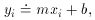where I use the symbol "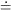" to represent the phrase "is fitted to." For the time being, we must assume that only the observable quantities yi contain observational errors; we must be certain that the quantities xi are known perfectly, or at least that the errors in the x's are negligible in comparison to the errors in the y's. If the observational errors in the quantities yi, which I will denote byi, follow the normal error distribution,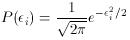(assuming that the standard error1),

wherethen the Likelihood,, of obtaining these fitting residuals is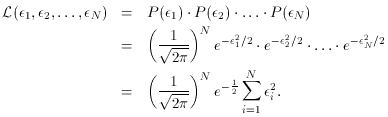This comes straight from elementary probability theory: if P(i) is the probability that the observed quantity y1 is wrong by an amounti, and P(2) is the probability that observed quantity y2 is wrong by amount2, and if the error that you made in y1 is independent of the error that you made in y2, then the probability of making errors1 and2 simultaneously is P(1) . P(2). This principle may be generalized to give the probability of observing the full set of observational errors1,2,...,N, which results in the equation forthat I just gave. The "method of maximum likelihood" seeks the values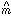and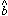for whichis maximized, since these are the most likely values of m and b, given the current set of data. (I use the "^" symbol to denote the specific estimate of a parameter which has been derived from the data by some statistical method; without the "^", the parameter is considered to be a variable). Clearly,is maximized when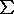i=1N,i2 is minimized: hence, for an assumed Gaussian error distribution, the method of maximum likelihood becomes the so-called "method of least squares." For this sum, we often use the symbolic representationThen,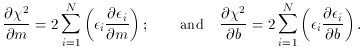2 is at a minimum and the Likelihood is at a maximum when:This meansFor the purposes of this discussion, it is convenient to write this pair of simultaneous equations as a matrix equation. Sincei=1N(1) = N we have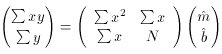so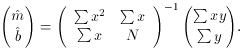And sincethe algebraic solution to this set of equations is:The quantity given byis called the mean error of unit weight, and is a very useful measure of the scatter of your data. It is essentially the root-mean-square value of your observational errors,i. (The -i are sometimes also referred to as the residuals of your yi values, but note that these are usually considered to have the opposite sign: residual = observation - model = yi - (mxi + b). Of course, when you square them, the sign makes no difference.) The sum of the squares of these residuals is divided by N - 2 instead of N to eliminate a bias in the estimate of the scatter: any two points define a straight line perfectly, so if there are two data points the residuals from the "best" fit will always be zero regardless of the true errors in the yi. It works similarly for other values of N: since we have used our data points to determine two previously unknown quantities, the observed scatter of the data about the best fit will always be a little bit less than the actual scatter about the true relationship. Dividing by N - 2 instead of N completely removes this bias; you can find a mathematical proof of this statement in a text on the theory of statistics.

If you had trouble following my derivation of the least-squares fit of a straight line, let's look at a couple of even simpler examples, to show how the general principle of minimizing the sum of the squares of the residuals can be used in real life. For the first example, let us assume that we have a set of observed values yi which are supposed to equal some constant. Of course, because of observational errors the various yi will not have a single, constant value, but instead will show some amount of scatter. Let's derive the least-squares estimator of that constant which "best" fits the observed yi. In the same notation as above, we want to solve a least-squares problem of the form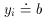where b is the desired constant. Here are the steps:In other words, the least-squares estimator of that constant parameter b which best fits some set of data yi is simply the arithmetic mean of those yi. This is why we have all grown up always taking the mean of a bunch of independent measurements: it can be shown mathematically to be the "best" way of extracting an estimate of some constant from error-prone data, provided that the observational errors follow a Gaussian distribution.

Now let's look at a slightly different problem. Suppose you know that your measured quantities yi should be linearly related to other quantities xi (which are known to infinite precision), but you also know that the best-fitting line must pass through the origin: y = 0 when x = 0. One astronomical example of this kind of problem is the calibration of the Hubble law for the expansion of the Universe: for some set of distant galaxies we measure the radial velocities of recession and estimate their distances, and then fit a straight line giving distance as a function of radial velocity (for the purposes of the present discussion, I assume that the radial velocities may be regarded as of infinite precision in comparison with the distances). Since our model assumes that the recession velocity must be zero at zero distance, we have to solve a problem of the form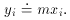Let's do it.This is the least-squares formula for determining the slope of a line which is forced to pass through the origin. It is just as fundamental as using the arithmetic mean to obtain the best estimate of a constant, but is less familiar to most of us.